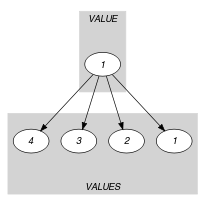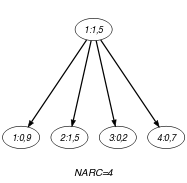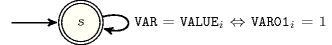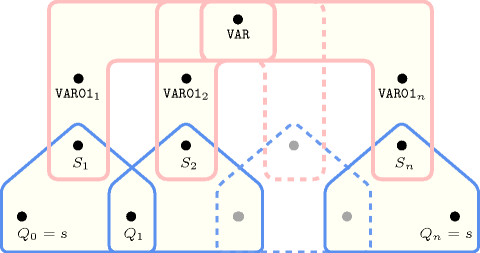## 5.136. domain_constraint

Origin
Constraint

$\mathrm{𝚍𝚘𝚖𝚊𝚒𝚗}_\mathrm{𝚌𝚘𝚗𝚜𝚝𝚛𝚊𝚒𝚗𝚝}\left(\mathrm{𝚅𝙰𝚁},\mathrm{𝚅𝙰𝙻𝚄𝙴𝚂}\right)$

Synonym

$\mathrm{𝚍𝚘𝚖𝚊𝚒𝚗}$.

Arguments
 $\mathrm{𝚅𝙰𝚁}$ $\mathrm{𝚍𝚟𝚊𝚛}$ $\mathrm{𝚅𝙰𝙻𝚄𝙴𝚂}$ $\mathrm{𝚌𝚘𝚕𝚕𝚎𝚌𝚝𝚒𝚘𝚗}\left(\mathrm{𝚟𝚊𝚛}\mathtt{01}-\mathrm{𝚍𝚟𝚊𝚛},\mathrm{𝚟𝚊𝚕𝚞𝚎}-\mathrm{𝚒𝚗𝚝}\right)$
Restrictions
 $\mathrm{𝚛𝚎𝚚𝚞𝚒𝚛𝚎𝚍}$$\left(\mathrm{𝚅𝙰𝙻𝚄𝙴𝚂},\left[\mathrm{𝚟𝚊𝚛}\mathtt{01},\mathrm{𝚟𝚊𝚕𝚞𝚎}\right]\right)$ $\mathrm{𝚅𝙰𝙻𝚄𝙴𝚂}.\mathrm{𝚟𝚊𝚛}\mathtt{01}\ge 0$ $\mathrm{𝚅𝙰𝙻𝚄𝙴𝚂}.\mathrm{𝚟𝚊𝚛}\mathtt{01}\le 1$ $\mathrm{𝚍𝚒𝚜𝚝𝚒𝚗𝚌𝚝}$$\left(\mathrm{𝚅𝙰𝙻𝚄𝙴𝚂},\mathrm{𝚟𝚊𝚕𝚞𝚎}\right)$
Purpose

Make the link between a domain variable $\mathrm{𝚅𝙰𝚁}$ and those 0-1 variables that are associated with each potential value of $\mathrm{𝚅𝙰𝚁}$: The 0-1 variable associated with the value that is taken by variable $\mathrm{𝚅𝙰𝚁}$ is equal to 1, while the remaining 0-1 variables are all equal to 0.

Example
$\left(\begin{array}{c}5,〈\begin{array}{cc}\mathrm{𝚟𝚊𝚛}\mathtt{01}-0\hfill & \mathrm{𝚟𝚊𝚕𝚞𝚎}-9,\hfill \\ \mathrm{𝚟𝚊𝚛}\mathtt{01}-1\hfill & \mathrm{𝚟𝚊𝚕𝚞𝚎}-5,\hfill \\ \mathrm{𝚟𝚊𝚛}\mathtt{01}-0\hfill & \mathrm{𝚟𝚊𝚕𝚞𝚎}-2,\hfill \\ \mathrm{𝚟𝚊𝚛}\mathtt{01}-0\hfill & \mathrm{𝚟𝚊𝚕𝚞𝚎}-7\hfill \end{array}〉\hfill \end{array}\right)$

The $\mathrm{𝚍𝚘𝚖𝚊𝚒𝚗}_\mathrm{𝚌𝚘𝚗𝚜𝚝𝚛𝚊𝚒𝚗𝚝}$ holds since $\mathrm{𝚅𝙰𝚁}=5$ is set to the value corresponding to the 0-1 variable set to 1, while the other 0-1 variables are all set to 0.

Typical
$|\mathrm{𝚅𝙰𝙻𝚄𝙴𝚂}|>1$
Symmetry

Items of $\mathrm{𝚅𝙰𝙻𝚄𝙴𝚂}$ are permutable.

Usage

This constraint is used in order to make the link between a formulation using finite domain constraints and a formulation exploiting 0-1 variables.

Reformulation

The $\mathrm{𝚍𝚘𝚖𝚊𝚒𝚗}_\mathrm{𝚌𝚘𝚗𝚜𝚝𝚛𝚊𝚒𝚗𝚝}$$\left(\mathrm{𝚅𝙰𝚁},$

$〈\mathrm{𝚟𝚊𝚛}\mathtt{01}-{B}_{1}\mathrm{𝚟𝚊𝚕𝚞𝚎}-{v}_{1},$

$\mathrm{𝚟𝚊𝚛}\mathtt{01}-{B}_{2}\mathrm{𝚟𝚊𝚕𝚞𝚎}-{v}_{2},$

$\cdots \cdots \cdots \cdots \cdots \cdots \cdots \cdots$

$\mathrm{𝚟𝚊𝚛}\mathtt{01}-{B}_{|\mathrm{𝚅𝙰𝙻𝚄𝙴𝚂}|}\mathrm{𝚟𝚊𝚕𝚞𝚎}-{v}_{|\mathrm{𝚅𝙰𝙻𝚄𝙴𝚂}|}〉\right)$

constraint can be expressed in term of the following reified constraint $\left(\mathrm{𝚅𝙰𝚁}={v}_{1}\wedge {B}_{1}=1\right)\vee \left(\mathrm{𝚅𝙰𝚁}={v}_{2}\wedge {B}_{2}=1\right)\vee \cdots \vee \left(\mathrm{𝚅𝙰𝚁}={v}_{|\mathrm{𝚅𝙰𝙻𝚄𝙴𝚂}|}\wedge {B}_{|\mathrm{𝚅𝙰𝙻𝚄𝙴𝚂}|}=1\right)$.

Systems
Keywords
Derived Collection
$\mathrm{𝚌𝚘𝚕}\left(\begin{array}{c}\mathrm{𝚅𝙰𝙻𝚄𝙴}-\mathrm{𝚌𝚘𝚕𝚕𝚎𝚌𝚝𝚒𝚘𝚗}\left(\mathrm{𝚟𝚊𝚛}\mathtt{01}-\mathrm{𝚒𝚗𝚝},\mathrm{𝚟𝚊𝚕𝚞𝚎}-\mathrm{𝚍𝚟𝚊𝚛}\right),\hfill \\ \mathrm{𝚒𝚝𝚎𝚖}\left(\mathrm{𝚟𝚊𝚛}\mathtt{01}-1,\mathrm{𝚟𝚊𝚕𝚞𝚎}-\mathrm{𝚅𝙰𝚁}\right)\right]\hfill \end{array}\right)$
Arc input(s)

$\mathrm{𝚅𝙰𝙻𝚄𝙴}$ $\mathrm{𝚅𝙰𝙻𝚄𝙴𝚂}$

Arc generator
$\mathrm{𝑃𝑅𝑂𝐷𝑈𝐶𝑇}$$↦\mathrm{𝚌𝚘𝚕𝚕𝚎𝚌𝚝𝚒𝚘𝚗}\left(\mathrm{𝚟𝚊𝚕𝚞𝚎},\mathrm{𝚟𝚊𝚕𝚞𝚎𝚜}\right)$

Arc arity
Arc constraint(s)
$\mathrm{𝚟𝚊𝚕𝚞𝚎}.\mathrm{𝚟𝚊𝚕𝚞𝚎}=\mathrm{𝚟𝚊𝚕𝚞𝚎𝚜}.\mathrm{𝚟𝚊𝚕𝚞𝚎}⇔\mathrm{𝚟𝚊𝚕𝚞𝚎𝚜}.\mathrm{𝚟𝚊𝚛}\mathtt{01}=1$
Graph property(ies)
$\mathrm{𝐍𝐀𝐑𝐂}$$=|\mathrm{𝚅𝙰𝙻𝚄𝙴𝚂}|$

Graph model

The $\mathrm{𝚍𝚘𝚖𝚊𝚒𝚗}_\mathrm{𝚌𝚘𝚗𝚜𝚝𝚛𝚊𝚒𝚗𝚝}$ constraint is modelled with the following bipartite graph:

• The first class of vertices corresponds to a single vertex containing the domain variable.

• The second class of vertices contains one vertex for each item of the collection $\mathrm{𝚅𝙰𝙻𝚄𝙴𝚂}$.

$\mathrm{𝑃𝑅𝑂𝐷𝑈𝐶𝑇}$ is used in order to generate the arcs of the graph. In our context it takes a collection with a single item $〈\mathrm{𝚟𝚊𝚛}\mathtt{01}-1\mathrm{𝚟𝚊𝚕𝚞𝚎}-\mathrm{𝚅𝙰𝚁}〉$ and the collection $\mathrm{𝚅𝙰𝙻𝚄𝙴𝚂}$.

The arc constraint between the variable $\mathrm{𝚅𝙰𝚁}$ and one potential value $v$ expresses the following:

• If the 0-1 variable associated with $v$ is equal to 1, $\mathrm{𝚅𝙰𝚁}$ is equal to $v$.

• Otherwise, if the 0-1 variable associated with $v$ is equal to 0, $\mathrm{𝚅𝙰𝚁}$ is not equal to $v$.

Since all arc constraints should hold the final graph contains exactly $|\mathrm{𝚅𝙰𝙻𝚄𝙴𝚂}|$ arcs.

Parts (A) and (B) of Figure 5.136.1 respectively show the initial and final graph associated with the Example slot. Since we use the $\mathrm{𝐍𝐀𝐑𝐂}$ graph property, the arcs of the final graph are stressed in bold.

##### Figure 5.136.1. Initial and final graph of the $\mathrm{𝚍𝚘𝚖𝚊𝚒𝚗}_\mathrm{𝚌𝚘𝚗𝚜𝚝𝚛𝚊𝚒𝚗𝚝}$ constraint(a) (b)
Signature

Since the number of arcs of the initial graph is equal to $\mathrm{𝚅𝙰𝙻𝚄𝙴𝚂}$ the maximum number of arcs of the final graph is also equal to $\mathrm{𝚅𝙰𝙻𝚄𝙴𝚂}$. Therefore we can rewrite the graph property $\mathrm{𝐍𝐀𝐑𝐂}$ $=$ $|\mathrm{𝚅𝙰𝙻𝚄𝙴𝚂}|$ to $\mathrm{𝐍𝐀𝐑𝐂}$ $\ge$ $|\mathrm{𝚅𝙰𝙻𝚄𝙴𝚂}|$. This leads to simplify $\underline{\overline{\mathrm{𝐍𝐀𝐑𝐂}}}$ to $\overline{\mathrm{𝐍𝐀𝐑𝐂}}$.

Automaton

Figure 5.136.2 depicts the automaton associated with the $\mathrm{𝚍𝚘𝚖𝚊𝚒𝚗}_\mathrm{𝚌𝚘𝚗𝚜𝚝𝚛𝚊𝚒𝚗𝚝}$ constraint. Let $\mathrm{𝚅𝙰𝚁}{\mathtt{01}}_{i}$ and ${\mathrm{𝚅𝙰𝙻𝚄𝙴}}_{i}$ respectively be the $\mathrm{𝚟𝚊𝚛}\mathtt{01}$ and the $\mathrm{𝚟𝚊𝚕𝚞𝚎}$ attributes of the ${i}^{th}$ item of the $\mathrm{𝚅𝙰𝙻𝚄𝙴𝚂}$ collection. To each triple $\left(\mathrm{𝚅𝙰𝚁},\mathrm{𝚅𝙰𝚁}{\mathtt{01}}_{i},{\mathrm{𝚅𝙰𝙻𝚄𝙴}}_{i}\right)$ corresponds a 0-1 signature variable ${S}_{i}$ as well as the following signature constraint: $\left(\left(\mathrm{𝚅𝙰𝚁}={\mathrm{𝚅𝙰𝙻𝚄𝙴}}_{i}\right)⇔\mathrm{𝚅𝙰𝚁}{\mathtt{01}}_{i}\right)⇔{S}_{i}$.

##### Figure 5.136.2. Automaton of the $\mathrm{𝚍𝚘𝚖𝚊𝚒𝚗}_\mathrm{𝚌𝚘𝚗𝚜𝚝𝚛𝚊𝚒𝚗𝚝}$ constraint##### Figure 5.136.3. Hypergraph of the reformulation corresponding to the automaton of the $\mathrm{𝚍𝚘𝚖𝚊𝚒𝚗}_\mathrm{𝚌𝚘𝚗𝚜𝚝𝚛𝚊𝚒𝚗𝚝}$ constraint: since all states variables ${Q}_{0},{Q}_{1},\cdots ,{Q}_{n}$ are fixed to the unique state $s$ of the automaton, the transitions constraints involve only a single variable and the constraint network is Berge-acyclic